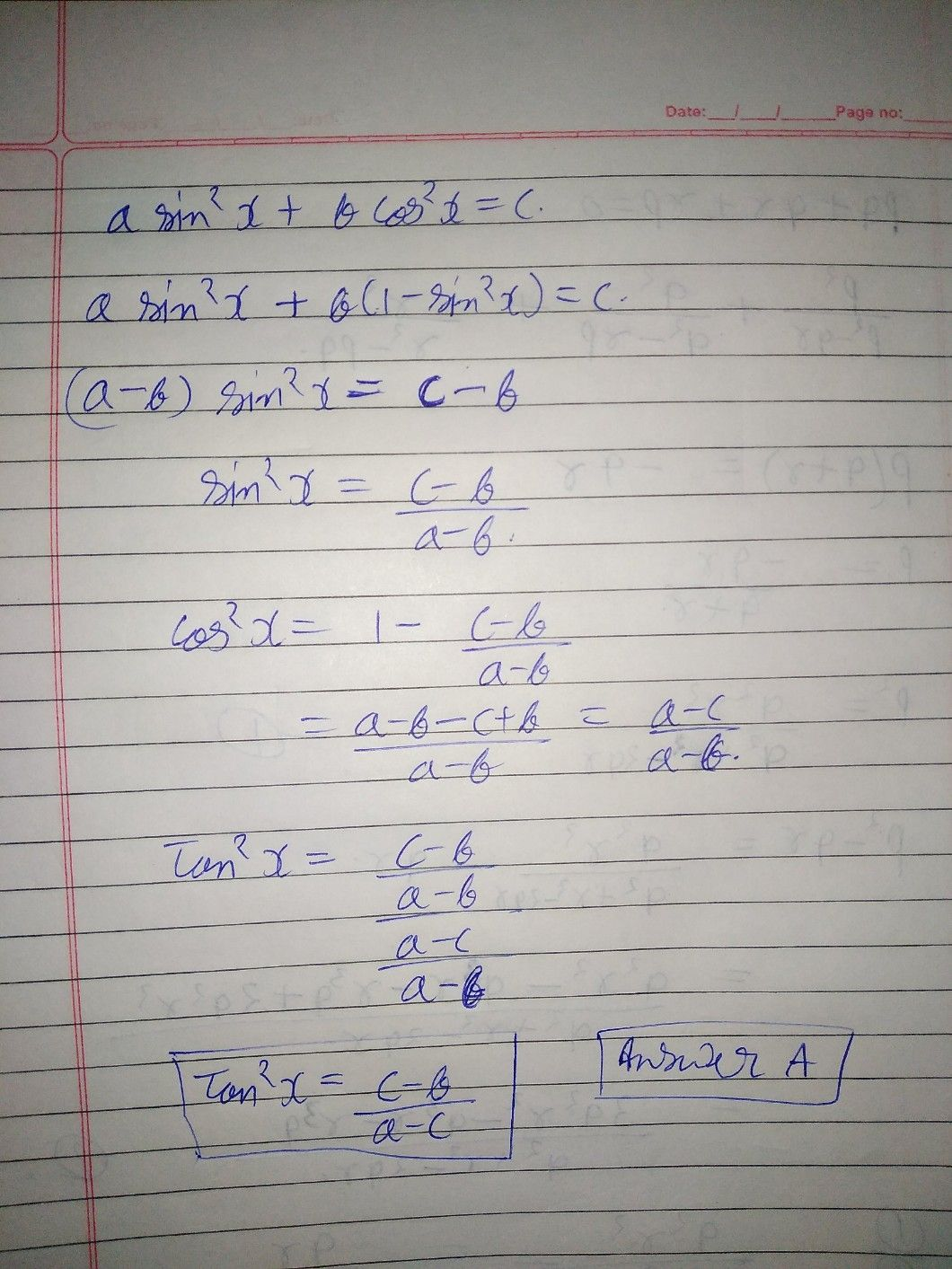Symbol
ProblemLet a $sin^{2}x+bcos^{2}x=c$ $bsin^{2}y+acos^{2}y=d$ and $p$ tan $nX=qtany$ $1.$ What is $tan^{2}x$ x equal to ? $\left(x\right)$ $\dfrac {c-b} {a-c}$ $\left(b\right)$ $\dfrac {a-c} {c-b}$ $yc0$ $\dfrac {c-a} {c-b}$ $\left(d\right)$ $\dfrac {c-b} {c-a}$